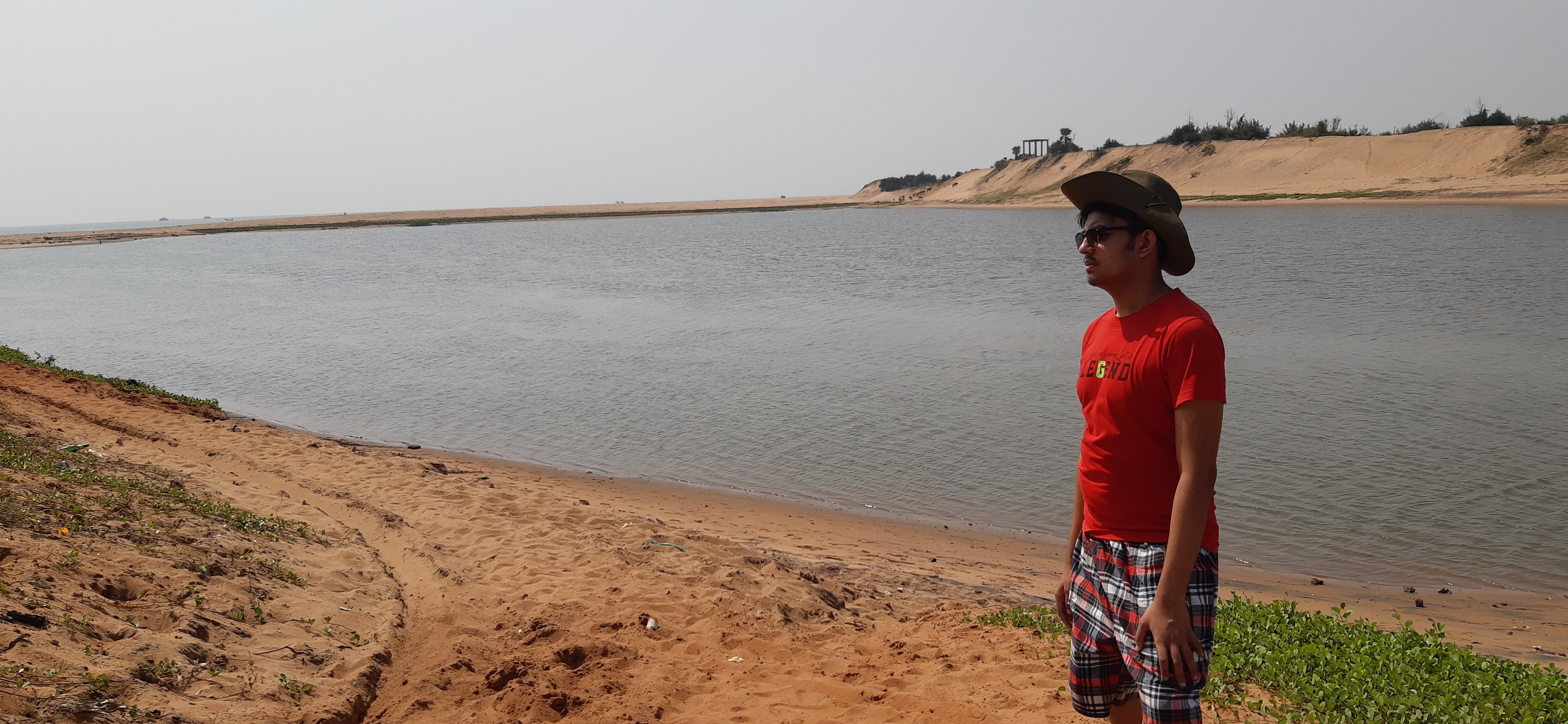Sriman Dutta

Senior Members

623

Everything posted by Sriman Dutta

1. @ TimeSpaceLightForce "All are golden eggs with varying weights" If so, then let us mark their masses as m1,m2,m3,...,m12. Out of these there are two such eggs that have the same mass. In such case, one option is to weight any two eggs and continue this for all the 12 golden eggs. The total possible combinations are 12!/2!(12-2)!=66. It's because if you pick one egg, you can weigh it with other 11 eggs. And this will happen for all 12 eggs, but there will be two identical combinations coming each case. Ex.- weighing m1 and m2, weighing m2 and m1. Thus, the final answer is 66. Hope it's correct.
2. Hi, I'm trying to find the area of a parachute cloth required for a landing. I should specify that it is not homework. Consider a person of mass m. Let the time required to come down to the surface safely be t. Let the height of the parachute be h. Then, h=1/2at^2, a=acceleration during the landing. As there will be much drag, using Newton's Second Law - Net force=Weight -Drag ma=mg-1/2dv^2CA Here, d=density of air, v=velocity of the parachute, C=drag coefficient and A=area of the parachute. So, it comes that - a=g-(dv^2CA)/2m Substituting the value of a in the first equation - h=1/2[g-(dv^2CA)/2m]t^2 Now to calculate the area required. The question is: Is my procedure correct?
3. Pass the light through such a material that has an unusually high refractive index. Then, it will be possible to overtake light in that material. LOL!!!
4. Actually permanent magnets are impossible. Magnets may demagnetise due to pressure, external magnetic fields, application of AC at its ends or due to chaotic alignment of the molecules of the magnet over time. In all cases, the ultimate consequence is that the magnet will lose its magnetic properties.
5. Hello, Suppose there's a circle with radius r and centre C. Let there be a point outside the circle P, such that the length PC is known. Now, lets take a point X on the circumference of the circle, such that the inclination of CX with respect to PC is a. Is it possible to find PX? If so, how??
6. :-)The glass is half filled with water. That means it is half filled with air too. Thus, the glass is full. Now, Clair says what is incorrect. So, she will say "it's empty".
7. Biophysics is an amalgam of biology and physics. It tends to use principles of physics to explain biological phenomenon. In today's world, there is a good scope of biophysics, it is applicable in medical research work, equipment development, genetic engineering and so on.
8. Such resonance and reverberation will alter the quality of sound. But, the OP says that the two guitars are identical.
9. COMPUTER - Commonly Operated Machine Practically Used for Technology, Education and Research Mouse - Manually Operated Utility Use for Selecting Equipments Virus - Valuable Information Resources Under Seize
10. Well, it's right that there is no relation between IQ and doing scientific things. And I won't like d*** here.
11. Ya, phase difference is a point, but it will not effect the quality of the sound produced. Consider the two guitar players in two different rooms, none can hear the other one. In such a case the quality of the note will be same.
12. Physics has gone through various stages, the earliest and the simplest being classical physics.
13. No, if and only if the guitars are exactly identical in shape and size, and also produces the same pitch.
14. Example of complex circuit.......(I think) a dynamo connected to a resistance through a step-up transformer. Moreover, for your information joe, electricity can only be transported through a circuit. Electricity is the major form of energy by which we can do almost all kinds of work by converting it to other forms of energy. (the law of conservation of energy) But, wait a circuit is not so cool.....Resistance will dissipate a lot of energy. Total energy dissipated it time t through a circuit of resistance R and current being I = I^2Rt
15. I don't see how mass can go from energy states to nuclear centre. The QM does have no law or principle which makes such weird things possible.
16. God and Ghosts are two words starting with same letter but are very much opposite in nature to the word Science.
×
×
• Create New...